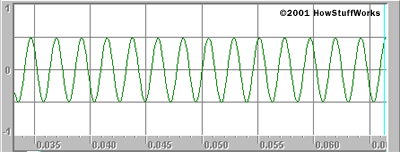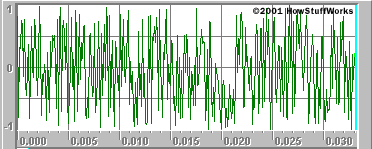# How Acoustic Guitars Work

## Sound, Tones, and Notes

The guitar is a musical instrument, so its goal in life is to make music. Music is the arrangement of tones into patterns that the human brain finds pleasing (or if not pleasing, then at least intriguing). In order to better understand music, let's start at the beginning: "What is sound?"

Sound is any change in air pressure that our ears are able to detect and process. For our ears to detect it, a change in pressure has to be strong enough to move the eardrums in our ears. The more strongly the pressure changes, the "louder" we perceive the sound to be.

For our ears to be able to perceive a sound, the sound has to occur in a certain frequency range. For most people, the range of perceivable sounds falls between 20 Hertz (Hz, oscillations per second) and 15,000 Hz. We cannot hear sounds below 20 Hertz or above 15,000 Hertz.

A tone is a sound that repeats at a certain specific frequency.

• Click here to hear a 440-Hz tone. (At the dialog select, click "Open.")
This 440-Hz tone can be pictured as a sine wave, like this:A tone is made up of one frequency or a very small number of related frequencies. The alternative to a tone is a combination of hundreds or thousands of random frequencies. We refer to these random-combination sounds as noise. When you hear the sound of a river, or the sound of wind rustling through leaves, or the sound of paper tearing or the sound made when you tune your TV to a nonexistent station, you are hearing noise.

• Click here to hear noise. (At the dialog select, click "Open")
Note: This is an unpleasant sound -- turn down your speakers before playing it.
Noise not only sounds random but also presents itself graphically as randomness:A musical note is a tone. However, a musical-note tone comes from a small collection of tones that are pleasing to the human brain when used together. For example, you might pick a set of tones at the following frequencies:

• 264 Hz
• 297 Hz
• 330 Hz
• 352 Hz
• 396 Hz
• 440 Hz
• 495 Hz
• 528 Hz

This particular collection of tones is known as the major scale. Each tone in the scale is multiplied by a certain fraction to come up with the next tone in the scale. Here's how the major scale works:

• 264 Hz * 9/8 = 297 Hz
• 297 Hz * 10/9 = 330 Hz
• 330 Hz * 16/15 = 352 Hz
• 352 Hz * 9/8 = 396 Hz
• 396 Hz * 10/9 = 440 Hz
• 440 Hz * 9/8 = 495 Hz
• 495 Hz * 16/15 = 528 Hz

Why are these particular fractions chosen in the major scale? Simply because they sound pleasing. Listen:

• Click here to hear the major scale. (At the dialog select, click "Open.")

These particular tones have been given letter names, and also word names, like this:

• 264 Hz - C, do (multiply by 9/8 to get:)
• 297 Hz - D, re (multiply by 10/9 to get:)
• 330 Hz - E, mi (multiply by 16/15 to get:)
• 352 Hz - F, fa (multiply by 9/8 to get:)
• 396 Hz - G, so (multiply by 10/9 to get:)
• 440 Hz - A, la (multiply by 9/8 to get:)
• 495 Hz - B, ti (multiply by 16/15 to get:)
• 528 Hz - C, do (multiply by 9/8 to get:)

And the sequence repeats.

The names are totally arbitrary, as with the fractions. It just turns out that they have a pleasing sound to human ears.

One thing to notice is that the two C notes are separated by exactly a factor of two -- 264 is one half of 528. This is the basis of octaves. Any note's frequency can be doubled to "go up an octave," and any note's frequency can be halved to "go down an octave."

You may have heard of "sharps" and "flats." Where do they come from? The scale of tones shown above is "in the key of C" because the fractions were applied with C as the starting note. If we were to start the fractions at D, with a frequency of 297, then we would be "tuned to the key of D" and the frequencies would look like this:

• 297 Hz, D, do (multiply by 9/8 to get:)
• 334.1 Hz, E, re (multiply by 10/9 to get:)
• 371.3 Hz, F, mi (multiply by 16/15 to get:)
• 396 Hz, G, fa (multiply by 9/8 to get:)
• 445.5 Hz, A, so (multiply by 10/9 to get:)
• 495 Hz, B, la (multiply by 9/8 to get:)
• 556.9 Hz, C, ti (multiply by 16/15 to get:)
• 594 Hz, D, do (multiply by 9/8 to get:)

And the sequence repeats.

The notes at 297 Hz (D), 396 Hz (G) and 495 Hz (B) in the key of D match the same notes in the key of C exactly. The E note in the key of D (at 334.1 Hz) is pretty close to the E note in the key of C (330 Hz). The same applies for the A note. F and C, however, are distinct in the two keys. F and C in the key of D are therefore referred to as F# (F sharp) and C# (C sharp) in the key of C. (Note that F sharp is also known as G flat, and C sharp is also known as D flat.) If you apply the fractions to several different keys, merge together all the identical and pretty-close notes and then look at the unique sharps that fall out, you realize that you need A#, C#, D#, F# and G# to handle all the keys.

You can see that, with all of these mergings of keys, the major scale can leave you with some pretty arbitrary decisions to make when you tune an instrument. For example, you can tune the major notes to the key of C, and then the sharps for F and C to the key of D, and the sharps for D and G to... It can get pretty messy.

Read on to learn how this problem was solved.